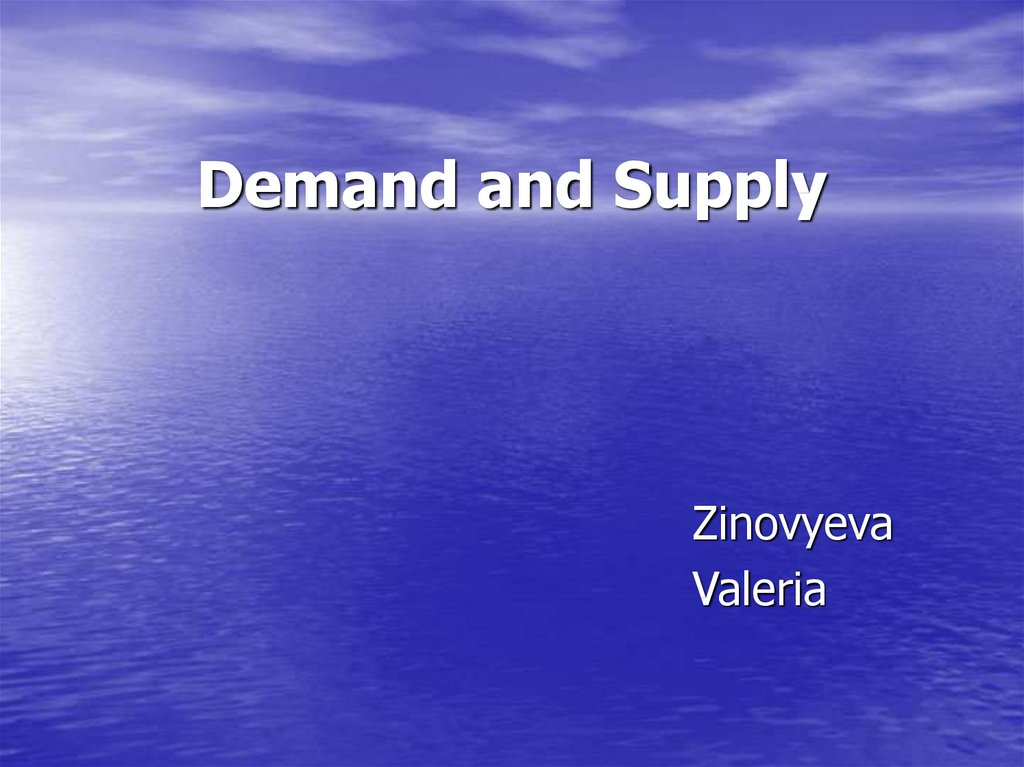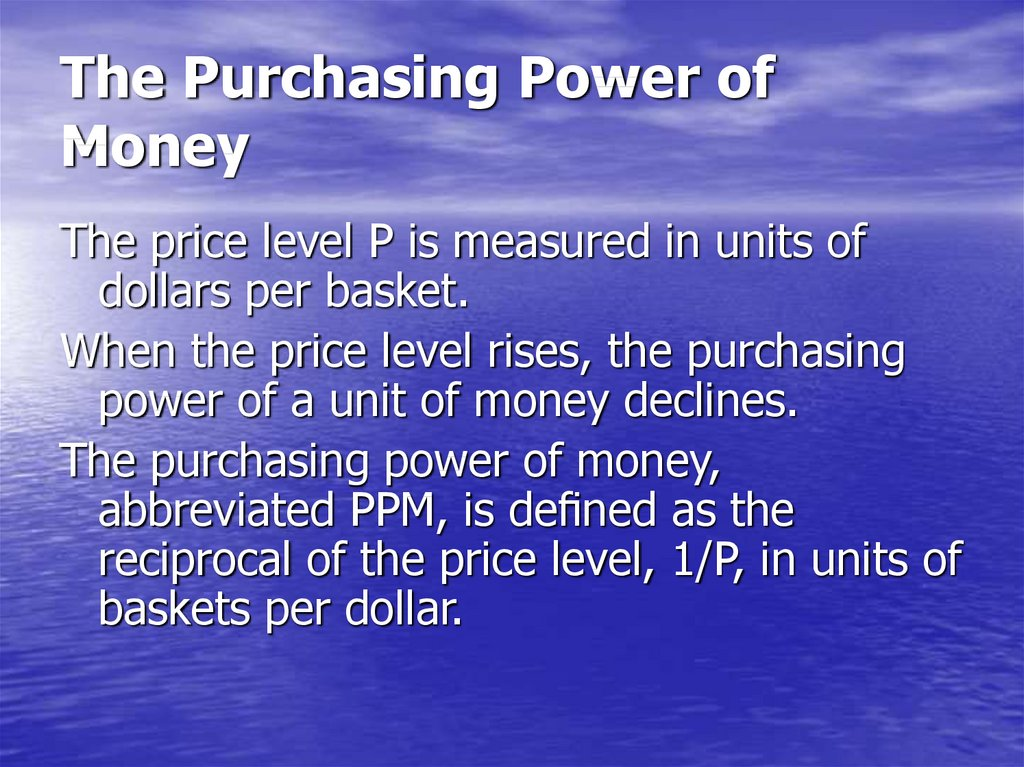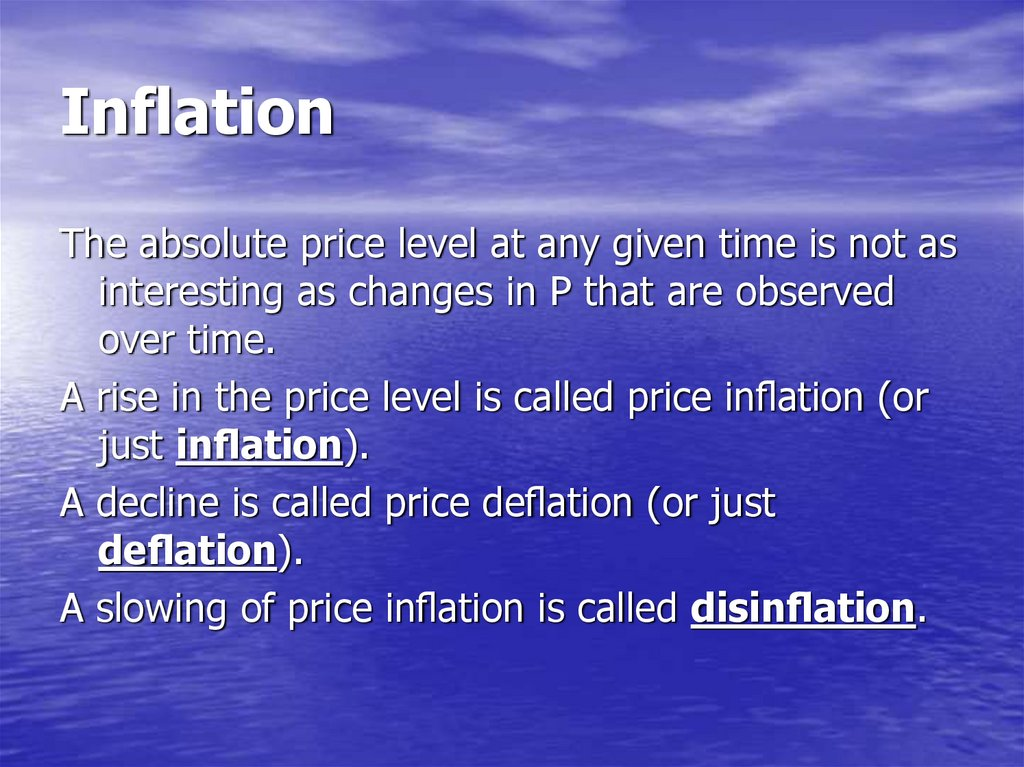# Demand and supply. The purchasing power of money

Zinovyeva
Valeria

## 2. The Purchasing Power of Money

The price level P is measured in units of
dollars per basket.
When the price level rises, the purchasing
power of a unit of money declines.
The purchasing power of money,
abbreviated PPM, is de ned as the
reciprocal of the price level, 1/P, in units of
baskets per dollar.

## 3. Inflation

The absolute price level at any given time is not as
interesting as changes in P that are observed
over time.
A rise in the price level is called price in ation (or
just in ation).
A decline is called price de ation (or just
de ation).
A slowing of price in ation is called disin ation.

## 4. Indices of Inflation

• Consumer Price Index (CPI)
• “producer price index” (PPI)
• “GDP de ator”
They generally correlate well with each
other

## 5. Consumer Price Index

- is a collection of goods and services
developed to replicate the spending
patterns of consumers.
The items in the CPI do not carry equal
weight but are assigned quantities that
are supposed to represent the
allocation of a typical consumer’s
income.

## 6. Producer price index

(PPI) purports to represent the costs faced
by typical producers

## 7. “GDP deﬂator”

“GDP de ator”
attempts to measure the prices of all the
goods and services produced in the entire
national economy, its “gross domestic
product.”

## 9. The Law of Demand for Money

The law of demand says that as the price of something
declines, people demand more of it, and this is true for
money.
Money’s purchasing power is its price, equal to the
reciprocal of the price level: PPM=1/P.
When the purchasing power of money declines (and the
price level rises) we want to hold more money, other
things being equal.
We desire money only because we can buy things with it,
and we need more to acquire the same goods and
services when money’s purchasing power declines.

## 10. Types of Demand for Money

The desire to acquire money to be spent
immediately (or in the near future) is
called transactions demand.
Money desired for expenditure later is a
form of saving, and is called portfolio
demand.

## 11. Transactions Demand for Money

First, people demand more money to hold
as the supply of goods and services
increases, other things being equal. As
wealth increases and if people want to
hold a constant proportion of their wealth
as money, then the amount of money they
want to hold must rise.

## 12.

Second in uence is the cost of acquiring
cash.
If acquiring cash requires standing in line at
a bank, people will keep larger amounts
on hand.
However, low-income people who lack bank
accounts and must rely on costly
commercial check-cashing businesses will
keep larger amounts of cash relative to
their monthly expenditures.

## 13. Third influence – the clearing system

Concerning electronic money…
Clearing system e ciencies reduce the
demand to hold money.

## 14. The degree of vertical integration among business ﬁrms

The degree of vertical
integration among business
rms
A forth and somewhat minor in uence on
the demand for money is the degree of
vertical integration among business rms.
After the acquisition, this same ow of
goods no longer required money as it
became simply a transfer of parts within
the newly integrated rm.

## 15. Portfolio Demand for Money

Money is the most liquid form of savings – you can
spend it immediately in case an emergency or
unexpected opportunity arises.
Money saved in a bank or other institution may
earn interest, but the rate is usually low.
One of the opportunity costs of holding money
is the higher interest you could probably earn on
less liquid forms of saving.

## 16. Opportunity cost of money

The demand for a good or service depends in part
on its price or cost.
The demand for a good or service depends in part
on its price or cost.
The cost of holding money is an opportunity cost
over time, because the alternative is investing
those funds to earn interest.
What one gets in return for giving up interest
income is the liquidity that money provides.
Each of us balances the opportunity cost of
holding money with the value of that liquidity.

## 17. One more classification of reasons for money demand There are three fundamental reasons as to why people demand money:

1) Transitory demand – the amount of money that you
require to settle transactions, since money is the
medium of exchange.
2) Precautionary demand – the amount of money required
in the event of unexpected need as a precautionary
store of liquidity.
3) Speculative demand – the amount of money required
for investment opportunities and / or luxury items.
People need money to reduce the riskiness of a portfolio
of assets by including some money in the portfolio,
since the value of money is very stable compared with
that of stocks, bonds, or real estate.

## 18. Demand for money

is the amount of money
that people desire to hold

## 19. Demand for money & income

Demand for money & income
The amount of money demanded by people
would change if their income increased.
They would demand more money (at a
given level of interest rates) primarily
because their transactions and
precautionary demands would increase at
their new higher level of spending.
An increase in their wealth would increase
their portfolio demand for money.

## 20. A households' demand for money primarily depends on:

• the interest rate,
• their income,
• and wealth,
• other variables.
Firms are also holders of money, in their
cash registers and bank accounts, for
essentially the same basic reasons as
households.

## 21. The demand for money can be represented by the following equation:

Md = k
×
P× y
Since the primary objective of money
demand is expenditure - money demand is
a function of expenditure (price × income)
k is a part of money, wanted to be held by
people

## 22. If income is zero then the demand for money is zero?

This is unrealistic because money demand is
required for survival (food).
So a more appropriate relationship for
money demand could be:
M d= a + k
× P × y where
a is an autonomous component of money
demand that is independent of the income
level.

## 23.

The demand for any good or service is
usually pictured in economics as a function
of its price, holding income and other
factors constant.
In the case of holding money, the "price" is
the opportunity cost of holding one dollar
for one year, the interest rate.
When we plot the quantity of money
demanded on the horizontal axis and the
interest rate on the vertical axis we will
have a demand curve.

## 25. Characteristics of Demand Curve

The quantity of money demanded is higher when the
interest rate is lower.
This inverse relationship between the interest rate and the
demand for money just reflects the fact that when the
opportunity cost of holding money is low, people will want
to hold more of it, and when it is high people will want to
hold less of it.
At very low levels of the interest rate the quantity of money
demanded increases dramatically, meaning that people
would then want to hold a very great amount of their
wealth in the form of money. Because the reward to
holding bonds is very small?
When the interest rate is very, very high, people will still
want to hold some money because it is even more costly
to revert to barter in making transactions.

## 27.

Rising wealth will contribute to higher demand for money
holdings through the portfolio motive.
Wealth would also roughly double in nominal terms over a
decade in which nominal income had doubled.
The quantity of money demanded at any given interest rate
will be much higher a decade later under our assumptions,
probably about twice its level a decade earlier.
This change in the demand for money depicted by shifting
the demand curve to the right. In Figure the doubling of
nominal incomes and wealth doubles the demand for
money at any given interest rate.
For example, at an interest rate of 5%, the quantity of
money demanded is \$1,200 billion at the end of the
decade, while it was only \$600 billion at the beginning of
the decade ago when nominal income and wealth were
half as great.

## 28. Demand for Money & Velocity

Demand for Money & Velocity
Since every dollar spent is someone’s dollar
of income, an overall drop in the demand
for money can only translate into
increased velocity, as we all spend faster.
Therefore the demand to hold money varies
inversely with velocity.

## 29. Demand for Money & Real Output

Demand for Money & Real Output
Another factor that in uences the demand
for money is real output, y.
When there are more goods and services
available for purchase, we tend to hold
more money.

## 30. Demand for Money & PPM

Demand for Money & PPM
Decline in the purchasing power of money
(i.e., a rise in P) leads to more demand for
money.

## 31. Equation of exchange

MV=PY
• the number of dollars (M)
• the average number of transactions (V )
• the price level (P)
• the real output (Y)

## 32. Units of Account

M is in dollars,
V is in transactions per year,
so MV is measured in dollars per year.
P is in dollars per basket
Y is in baskets per year
so PY is also in dollars per year.

## 33.

Let's put together a simple economy of
four people where each person has the
following:
Person
Endowment
1
\$100 in currency
2
2 tickets worth \$50 each
3
\$100 calculator
4
25 drawing pencil worth \$4 each

## 34.

Suppose the following transactions take place:
Person 1 wants a calculator, so she trades her \$100
in currency to person 3 for the calculator.
Person 3 wants the tickets so she takes the \$100 in
currency from person 1 and gives it to person 2 for
the tickets.
Person 2, who now has the \$100, needs the
drawing pencils and gives the \$100 to person 4 for
the pencils.
The total value of the transactions was:
\$100 ¤ (1 calculator) + \$50 ¤ (2 tickets) + \$4 ¤ (25
pencils) = \$300

## 35.

In this economy, the \$100 in currency was used
three times and generated \$300 worth of
transactions.
(# of Dollars) ¤ (Times Used) = Value of Transactions
\$100
M
¤
¤
3
V
\$300
= Nominal GDP
=
Velocity of money - the number of times the
dollars were used.

## 36.

M
¤
V
= Nominal GDP
Nominal GDP = Price Level *Real GDP
Nominal GDP =
P
¤
Y
MV = PY
The equation of exchange says that the
quantity of money multiplied by its
velocity equals the level of nominal GDP
written as the price level multiplied by real
GDP.

## 37.

According to Irving Fisher
MV=P Q1 +
MV=P2Q2 +
MV=P3Q3 +
1
Thus,
MV=∑PQ
etc.

## 38. The Quantity Theory of Money

The quantity theory of money states that
money growth translates directly into
inflation.
In the short run %∆V = 0, %∆Y = 0
Thus
%∆M = %∆P

## 39.

The equation explains why money growth
exceed inflation in low-inflation economies
The money growth can be partly absorbed because
these countries are seeing real growth.
Let us suppose that a country has 5% money
growth, constant velocity, and 3% real growth.
Thus the equation of exchange can predict the
inflation rate. We find
5 + 0 = %∆P + 3
%∆P = 2
We would expect inflation to be 2% which is clearly
less than money growth.

## 40.

Individuals require money to complete
transactions which means that:
the number of dollars needed equals the
total dollar value of transactions divided
by the number of times each dollar was
used

## 41. The Supply of Money

• is the quantity of money (currency and bank
deposits) set by the central bank
• is the number of currency units available to be
offered by a person for credit
Measured by total amount of money limited by
central bank of a state.
Depends on central bank’s decision.
Measured by amount of money which can be
offered by a person for credit.

## 42. Supply of Money depends on:

• The reserve requirement
• Total amount of refinancing made by
central bank
• Level of wealth of the society
• Transparency of enterprising
• Level of bank credibility

## 43. The reserve requirements

(or cash reserve ratio) is a central bank
regulation that sets the minimum reserves
each commercial bank must hold to
customer deposits and notes.
The reserve ratio is sometimes used as a
tool in the monetary policy, influencing the
country's economy, borrowing, and
interest rates.

## 44. Characteristics of Supply Curve

Since the supply of money does not vary
with the rate of interest, the supply curve
of money simply can be depicted as a
vertical line at the actual quantity of
money.
The "price" of money is the interest rate at
which the demand for money equals the
supply of money.

## 46. Balance of supply & demand

Balance of supply & demand
In Figure the supply of money is a vertical line at the
quantity \$300 billion, indicating that in this
hypothetical economy the central bank has set the
supply of money at \$300 billion.
The supply of money is fixed at that quantity, and it
will remain there until the central bank decides to
change it.
The quantity of money demanded is equal to the
quantity supplied, \$300 billion, at an interest rate of
10%. At that interest rate, people are content to
hold the quantity of money that is supplied by the
central bank.

## 47. If there is no balance of S&D…

If there is no balance of S&D…
If the interest rate is 9% instead of 10%. At an opportunity cost of
only 9% people would want to hold more money than when the
opportunity cost is 10%. As we see in Figure, they would want to
hold more money than the central bank has actually supplied. In an
attempt to increase their holdings of money to the level they desire,
people would sell some of their bonds in order to increase their
holdings of money.
While any one economic agent can increase or decrease the quantity of
money that they hold, all economic agents taken together cannot
change the quantity of money that they hold because the quantity
of money is fixed.
Everybody try to sell bonds to increase their holding of money - bond
yields begin to rise. The interest rate is just the yield on bonds, we
see that the interest rate would rise and it would continue to rise
until it was back up to 10%. At that point the interest rate would
stop rising because people would be content to hold the quantity of
money that exists.

## 48. A Shift in the Supply of Money

Imagine that the central bank boosts the money
supply suddenly from \$300 billion to \$600 billion. In
Figure this is indicated by the shift in the vertical
supply line to the right.
At the old interest rate of 10%, agents wish to hold
only \$300 billion, not \$600 billion.
At an interest rate of 5% the quantity of money
demanded is equal to the supply, \$600 billion.
Money is now much cheaper to hold because there
is much more of it available.
Finally, at an interest rate of 5% the agents in this
economy are content to hold the \$600 billion supply
of money.

## 50. Central Bank Increases Supply of Money

When the central bank buys bonds in an open market
operation, it increases the supply of money & it causes
the "price" or opportunity cost of holding money to fall.
Thus, by increasing the supply of money the central bank
can push the interest rate down, and by reducing the
supply of money it can push interest rates up.
Lower interest rates stimulate investment spending on new
plant and equipment by business and spending on
durable goods by households because the cost of
borrowing has fallen.
The result is higher production and higher employment, at
least until prices and wages adjust to the increase in
demand. If the central bank persists in more rapid
expansion of the money supply then inflation will
accelerate.

## 51. Central Bank Reduces Supply of Money

When the central bank sells bonds it reduces the
money supply. Smaller supply means higher
price, in this case higher interest rates.
Higher interest rates mean that some investment
projects are not undertaken and some houses
are not built that would have been otherwise
because loans are more costly. Demand for
goods in the economy then falls, and with it
production and employment. Inflation will
decline.

## 52.

The supply of money (Ms) is
determined by the central bank and
the banking sector. Equilibrium would
require that money supply equals
money demand
Md = Ms